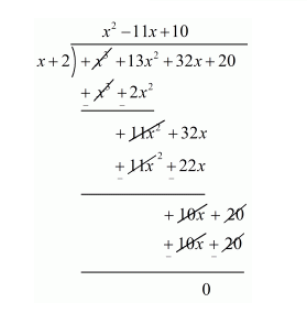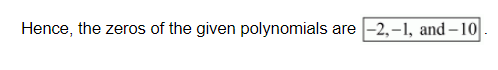# Obtain all zeros of f(x) = x3 + 13x2 + 32x + 20,

Question:

Obtain all zeros of $1(x)=x^{3}+13 x^{2}+32 x+20$, if one of its zeros is $-2$.

Solution:

Since $-2$ is one zero of $f(x)$.

Therefore, we know that, if $x=\alpha$ is a zero of a polynomial, then $(x-\alpha)$ is a factor of $f(x)=x+2$ is a factor of $f(x)$.

Now, we divide $f(x)=x^{3}+13 x^{2}+32 x+20$ by $g(x)=(x+2)$ to find the others zeros of $f(x)$.By using that division algorithm we have, $f(x)=g(x) \times q(x)+r(x)$

$x^{3}+13 x^{2}+32 x+20=(x+2)\left(x^{2}+11 x+10\right)+0$

$x^{3}+13 x^{2}+32 x+20=(x+2)\left(x^{2}+10 x+1 x+10\right)$

$x^{3}+13 x^{2}+32 x+20=(x+2)[x(x+10)+1(x+10)]$

$x^{3}+13 x^{2}+32 x+20=(x+2)(x+1)(x+10)$